# Oops...

This website is made for modern browsers. You are seeing this, because your browser is missing a feature or two. Please install the latest update or switch to a modern browser. See you soon.

# Canvas search

#UI

Grasshopper offers a search function that gives us quick access to all available components. Double-clicking on an empty space on the canvas or hitting Space opens a search box that lets us find components by keywords. Once we are familiar with the components' names, this method provides a faster flow than hunting for icons in the tabs.

After starting to type, the search dialog suggests components that match our input by scanning all components' names and nicknames. The nickname can be found (if there is any) by hovering a component (see figure below). For example, by typing ccx we can find the component Curve | CurveCurve | Curve (CCX)
Intersect  >  Physical  >  Curve | Curve
Solve intersection events for two curves.
Inputs
Curve A (A)First curve
Curve B (B)Second curve
Outputs
Points (P)Intersection events
Params A (tA)Parameters on first curve
Params B (tB)Parameters on second curve
. After hitting Enter, the selected component is placed on the canvas.

Besides using the predefined nicknames, we can set a personal Component Alias which will then also be searched for. For this, we right-click the desired component in the ribbon tab and then assign a custom Component Alias.

### Special shortcuts

Some components can be invoked with special shortcuts and thus, are not placed on the canvas with default values but can be preconfigured through the canvas search. For example, when we begin our query with a quotation mark, a PanelPanel
Params  >  Input  >  Panel
A panel for custom notes and text values
is creating and its content is set to whatever followed the quotation mark: "Hello becomes a panel with Hello.

The following shortcuts can be used within the search dialog:

ShortcutFunction
"abcCreates a PanelPanel
Params  >  Input  >  Panel
A panel for custom notes and text values
with content abc.
//abcCreates a PanelPanel
Params  >  Input  >  Panel
A panel for custom notes and text values
with content abc.
<123Creates Smaller Than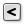Smaller Than (Smaller)
Maths  >  Operators  >  Smaller Than
Smaller than (or equal to)
Inputs
First Number (A)Number to test
Second Number (B)Number to test against
Outputs
Smaller than (<)True if A < B
… or Equal to (<=)True if A <= B
and input B is set to 123.
>123Creates Larger Than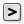Larger Than (Larger)
Maths  >  Operators  >  Larger Than
Larger than (or equal to)
Inputs
First Number (A)Number to test
Second Number (B)Number to test against
Outputs
Larger than (>)True if A > B
… or Equal to (>=)True if A >= B
and input B is set to 123.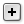Addition (A+B)
Inputs
Outputs
and input B is set to 123.
-123Creates Subtraction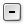Subtraction (A-B)
Maths  >  Operators  >  Subtraction
Mathematical subtraction
Inputs
A (A)First operand for subtraction
B (B)Second operand for subtraction
Outputs
Result (R)Result of subtraction
and input B is set to 123.
*123Creates Multiplication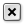Multiplication (A×B)
Maths  >  Operators  >  Multiplication
Mathematical multiplication
Inputs
A (A)First item for multiplication
B (B)Second item for multiplication
Outputs
Result (R)Result of multiplication
and input B is set to 123.
/123Creates Division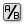Division (A/B)
Maths  >  Operators  >  Division
Mathematical division
Inputs
A (A)Item to divide (dividend)
B (B)Item to divide with (divisor)
Outputs
Result (R)The result of the Division
and input B is set to 123.
\123Creates Integer Division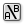Integer Division (A\B)
Maths  >  Operators  >  Integer Division
Mathematical integer division
Inputs
A (A)Item to divide (dividend)
B (B)Item to divide with (divisor)
Outputs
Result (R)Result of integer division
and input B is set to 123.
=123Creates Equality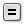Equality (Equals)
Maths  >  Operators  >  Equality
Test for (in)equality of two numbers
Inputs
First Number (A)Number to compare
Second Number (B)Number to compare to
Outputs
Equality (=)True if A = B
Inequality (≠)True if A ≠ B
and input B is set to 123.
%123Creates Modulus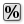Modulus (Mod)
Maths  >  Operators  >  Modulus
Divides two numbers and returns only the remainder.
Inputs
A (A)First number for modulo (dividend)
B (B)Second number for modulo (divisor)
Outputs
Result (R)The remainder of A/B
and input B is set to 123.
&abcCreates Concatenate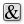Concatenate (Concat)
Sets  >  Text  >  Concatenate
Concatenate some fragments of text
Inputs
Fragment A (A)First text fragment
Fragment B (B)Second text fragment
Outputs
Result (R)Resulting text consisting of all the fragments
and input B is set to abc.
!Creates Explode Tree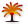Explode Tree (BANG!)
Sets  >  Tree  >  Explode Tree
Extract all the branches from a tree
Inputs
Data (D)Data to explode
Outputs
Branch 0 (-)All data inside the branch at index: 0
Branch 1 (-)All data inside the branch at index: 1
.
~abcCreates a Scribble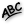Scribble (Scribble)
Params  >  Util  >  Scribble
A quick note
with content abc.
1,2,3Creates a Point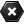Point (Pt)
Params  >  Geometry  >  Point
Contains a collection of three-dimensional points
with coordinates 1,2,3.
123Creates a Number Slider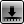Number Slider
Params  >  Input  >  Number Slider
Numeric slider for single values
preset to the value 123. Steps size are integers.
1.23Creates a Number SliderNumber Slider
Params  >  Input  >  Number Slider
Numeric slider for single values
preset to the value 1,23. Slider accuracy correlates with the decimal places.
1<5Creates a Number SliderNumber Slider
Params  >  Input  >  Number Slider
Numeric slider for single values
with a numeric domain from 1 to 5 and presets the value to 1. Steps size are integers.
1..5All Number SliderNumber Slider
Params  >  Input  >  Number Slider
Numeric slider for single values
shortcuts can also be written with two .. instead of <.
1.0<5Creates a Number SliderNumber Slider
Params  >  Input  >  Number Slider
Numeric slider for single values
with a numeric domain from 1.0 to 5.0 and presets the value to 1.0. Slider accuracy correlates with the decimal places.
1<3<5Creates a Number SliderNumber Slider
Params  >  Input  >  Number Slider
Numeric slider for single values
with a numeric domain from 1 to 5 and presets the value to 3. Steps size are integers.
1.0<3<5Creates a Number SliderNumber Slider
Params  >  Input  >  Number Slider
Numeric slider for single values
with a numeric domain from 1.0 to 5.0 and presets the value to 3.0. Slider accuracy correlates with the decimal places.

Even though input B can be set directly on some components, it’s highly recommended to not use this shortcut, but to assign the value later with another component. Internally set values are much harder to debug later and thus compromise the readability of our algorithm. Nevertheless, the shortcut still creates the desired component even if no value is provided.

This page is open source. Edit it on GitHub or see how you can contribute.